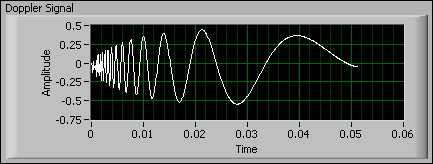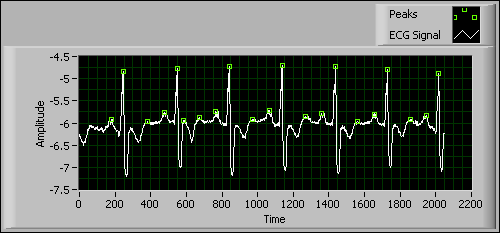# Introduction to Wavelet Signal Processing (Advanced Signal Processing Toolkit)

LabVIEW 2014 Advanced Signal Processing Toolkit Help

Edition Date: June 2014

Part Number: 372656C-01

»View Product InfoDownload Help (Windows Only)

Wavelets are functions that you can use to decompose signals. Just as the Fourier transform decomposes a signal into a family of complex sinusoids, the wavelet transform decomposes a signal into a family of wavelets. Unlike sinusoids, which are symmetric, smooth, and regular, wavelets can be either symmetric or asymmetric, sharp or smooth, regular or irregular. The following figure compares a sine wave with the db02 wavelet and the FBI wavelet.In previous figure, you can see that the Sine Wave is symmetric, smooth, and regular. The db02 Wavelet is asymmetric, sharp, and irregular. The FBI Wavelet is symmetric, smooth, and regular. You also can see that a sine wave has an infinite length, whereas a wavelet has a finite length.

For different types of signals, you can select different types of wavelets that best match the features of the signal you want to analyze. Therefore, you can perform wavelet signal processing and generate reliable results about the underlying information of a signal.

The family of wavelets contains the dilated and translated versions of a prototype function. Traditionally, the prototype function is called a mother wavelet. The scale and shift of wavelets determine how the mother wavelet dilates and translates along the time or space axis. A scale factor greater than one corresponds to a dilation of the mother wavelet along the horizontal axis, and a positive shift corresponds to a translation to the right of the scaled wavelet along the horizontal axis. The following figure shows the db02 mother wavelet and the associated dilated and translated wavelets with different scale factors and shift values.## Wavelet Transform

The wavelet transform computes the inner products of a signal with a family of wavelets. The wavelet transform tools are categorized into continuous wavelet tools and discrete wavelet tools. Usually, you use the continuous wavelet tools for signal analysis, such as self-similarity analysis and time-frequency analysis. You use the discrete wavelet tools for both signal analysis and signal processing, such as noise reduction, data compression, peak detection and so on.

In contrast with sinusoids, wavelets are localized in both the time and frequency domains, so wavelet signal processing is suitable for nonstationary signals, whose spectral content changes over time. The adaptive time-frequency resolution of wavelet signal processing enables you to perform multiresolution analysis on nonstationary signals. The properties of wavelets and the flexibility to select wavelets make wavelet signal processing a beneficial tool for feature extraction applications.

## Benefits of Wavelet Signal Processing

Wavelet signal processing is different from other signal processing methods because of the unique properties of wavelets. For example, wavelets are irregular in shape and finite in length. Wavelet signal processing can represent signals sparsely, capture the transient features of signals, and enable signal analysis at multiple resolutions.

### Sparse Representation

Wavelets are localized in both the time and frequency domains because wavelets have limited time duration and frequency bandwidth. The wavelet transform can represent a signal with a few coefficients because of the localization property of wavelets. The following figure shows the waveform of the Doppler signal.The following figure shows the discrete wavelet transform (DWT) coefficients of the Doppler signal.In the previous figure, most of the DWT coefficients are zero, which indicates that the wavelet transform is a useful method to represent signals sparsely and compactly. Therefore, you usually use the DWT in some signal compression applications.

### Transient Feature Detection

Transient features are sudden changes or discontinuities in a signal. A transient feature can be generated by the impulsive action of a system and frequently implies a causal relationship to an event. For example, heartbeats generate peaks in an electrocardiogram (ECG) signal.

Transient features generally are not smooth and are of short duration. Because wavelets are flexible in shape and have short time durations, the wavelet signal processing methods can capture transient features precisely. The following figure shows an ECG signal and the peaks detected with the wavelet transform-based method. This method locates the peaks of the ECG signal precisely.Refer to the ECG QRS Complex Detection VI in the labview\examples\Wavelet Analysis\WAApplications directory for an example of wavelet transform-based peak detection in an ECQ signal.

The LabVIEW Wavelet Analysis Tools provide many types of wavelets, such as the Daubechies, Haar, and Coiflet wavelets.

### Multiple Resolutions

Signals usually contain both low-frequency components and high-frequency components. Low-frequency components vary slowly with time and require fine frequency resolution but coarse time resolution. High-frequency components vary quickly with time and require fine time resolution but coarse frequency resolution. You need to use a multiresolution analysis (MRA) method to analyze a signal that contains both low- and high-frequency components.

Wavelet signal processing is naturally an MRA method because of the dilation process. The following figure shows the wavelets with different dilations and their corresponding power spectra.The Wavelets graph contains three wavelets with different scales and translations. The Power Spectra of Wavelets graph shows the power spectra of the three wavelets, where a and u represent the scale and shift of the wavelets, respectively. The previous figure shows that a wavelet with a small scale has a short time duration, a wide frequency bandwidth, and a high central frequency. This figure also shows that a wavelet with a large scale has a long time duration, a narrow frequency bandwidth, and a low central frequency.

The time duration and frequency bandwidth determine the time and frequency resolutions of a wavelet, respectively. A long time duration means coarse time resolution. A wide frequency bandwidth means coarse frequency resolution. The following figure shows the time and frequency resolutions of the three wavelets with three boxes in the time-frequency domain. The heights and widths of the boxes represent the frequency and time resolutions of the wavelets, respectively. This figure shows that a wavelet with a small scale has fine time resolution but coarse frequency resolution and that a wavelet with a large scale has fine frequency resolution but coarse time resolution.The fine frequency resolution of large-scale wavelets enables you to measure the frequency of the slow variation components in a signal. The fine time resolution of small-scale wavelets enables you to detect the fast variation components in a signal. Therefore, wavelet signal processing is a useful multiresolution analysis tool.